Courses

# RD Sharma Solutions - Ex - 9.2, Ratio And Proportion, Class 7, Math Class 7 Notes | EduRev

## RD Sharma Solutions for Class 7 Mathematics

Created by: Abhishek Kapoor

## Class 7 : RD Sharma Solutions - Ex - 9.2, Ratio And Proportion, Class 7, Math Class 7 Notes | EduRev

The document RD Sharma Solutions - Ex - 9.2, Ratio And Proportion, Class 7, Math Class 7 Notes | EduRev is a part of the Class 7 Course RD Sharma Solutions for Class 7 Mathematics.
All you need of Class 7 at this link: Class 7

#### Question 1:

Which ratio is larger in the following pairs?
(i) 3 : 4 or 9 : 16
(ii) 15 : 16 or 24 : 25
(iii) 4 : 7 or 5 : 8
(iv) 9 : 20 or 8 : 13
(v) 1 : 2 or 13 : 27

(i) Writing the ratios as fractions, we have
3 : 4 = 3/4 and 9 : 16 = 9/16
Now, LCM of 4 and 16 = 16.
Making the denominator of each fraction = 16, we have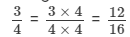and the other fraction = 9/16

Of 12/16 and 9/16,  clearly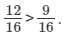therefore,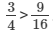Writing the ratios as fractions, we have
15 : 16 = 15/16 and 24 : 25 = 24/25
Now, LCM of 16 and 25 = 400.
Making the denominator of each fraction = 400, we have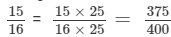and the other fraction =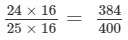Clearly, 384 > 375. So,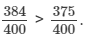Therefore,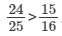(iii) Writing the ratios as fractions, we have
4 : 7 = 4/7 and 5 : 8 = 5/8
Now, LCM of 7 and 8 = 56.
Making the denominator of each fraction = 56, we have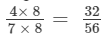and the other fraction =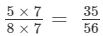Clearly, 36 > 32. So,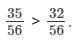Therefore,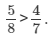(iv) Writing the ratios as fractions, we have
9 : 20 = 9/20 and 8 : 13 = 8/13
Now, LCM of 20 and 13 = 260.
Making the denominator of each fraction = 260, we have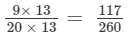and the other fraction =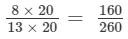Clearly, 160 > 117. So,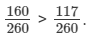Therefore,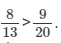(v) Writing the ratios as fractions, we have
1 : 2 = 1/2 and 13 : 27 = 13/27
Now, LCM of 2 and 27 = 54.
Making the denominator of each fraction = 54, we have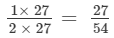and the other fraction =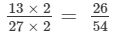Clearly, 27 > 26. So,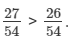Therefore,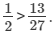#### Question 2:

Give two equivalent ratios of 6 : 8.

We have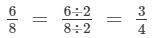Therefore, 3 : 4 is an equivalent ratio of 6 : 8.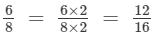Hence, 3 : 4 and 12 : 16 are equivalent ratios of 6 : 8.

#### Question 3:

Fill in the following blanks: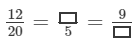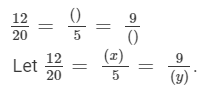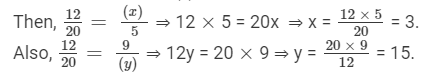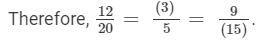97 docs

,

,

,

,

,

,

,

,

,

,

,

,

,

,

,

,

,

,

,

,

,

,

,

,

,

,

,

,

,

,

;